# Right Angles

Right Angles• Right angles are exactly 90 .
• This means that the two lines that form a right angle will meet to form a capital ‘L’ shape.
• The lines that form the angle will line up directly with the sides of a ruler.
• To identify a right angle, place a ruler or set-square in the corner of the angle and see if the sides line up.
• If the angle doesn’t line up with the sides of the ruler or the set square then it is not a right angle.
• We draw a square in the corner of the angle to show that it is a right angle.
• We look out for and lines that may meet at right angles.• We will test the angles of this triangle with a ruler to see if any of them are right angles.
• The sides of the ruler line up perfectly with the angle in the bottom left corner and so, it is a right-angle.
• We mark right angles with a square.
• We place the ruler along the sides of the other angles but neither of them line up.
• We only have one right angle.
• We call a triangle that contains a right angle a right-angled triangle.

The lines that make right angles line up with the sides of a ruler or set square.

We mark right angles with a small square in the corner of the angle.• We line the ruler up with each angle in our trapezium.
• We can see that the two base angles are right angles because the sides line up with the sides of the ruler.
• We mark the two right angles with a square in each angle.
• The other two angles in this shape are not right angles because the ruler does not line up with both sides of each angle.Supporting Lessons# Right Angles

## What are Right Angles?

A right angle is a special type of angle that contains exactly 90 degrees. It is one quarter of a full turn. Right angles are found in all four corners of a square. We mark right angles with a square in the corner of the angle.

Right angles are always formed by any two horizontal and vertical lines.We mark the right angle with a square in the corner of the angle. Any time we see this square, we know we have a right angle.

Here is a right angle shown on a protractor. We can see that the lines are horizontal and vertical.Right angles are the angles found in the corners of squares or rectangles. Right angles look like capital ‘L’ shapes.

Here are some examples of right angles found in real life.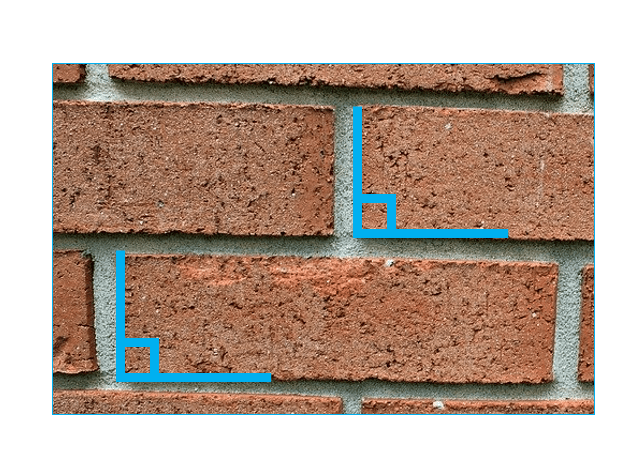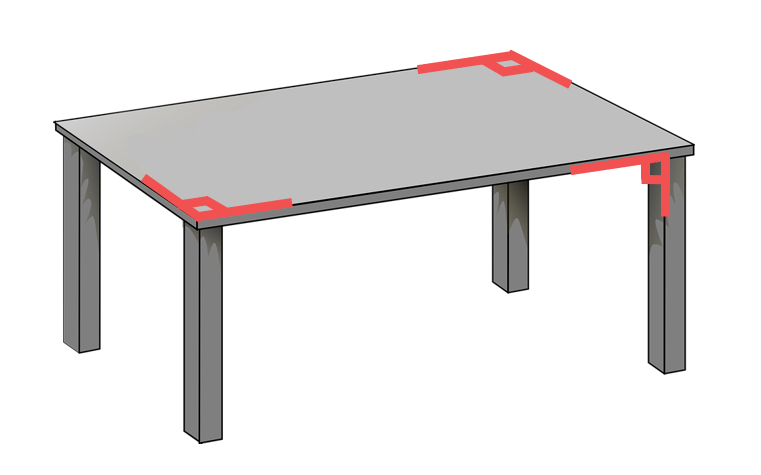In shapes, right angles are often made up of one

line and one line.

## How to Check if an Angle is a Right Angle

To test if an angle is right angle, place a protractor over one line of the angle and read the number that is in line with the other line of the angle. If the angle is 90 degrees, then it is a right angle.

It can be easier to place the corner of a ruler or set-square in the corner of an angle and check if both sides of the angle line up with the sides of the ruler or the set square.

The angle in the corner of a ruler is a right angle so if the sides of the ruler line up exactly with both sides of the angle, then the angle itself is a right angle.

If both sides of the angle do not line up with the sides of the ruler, then the angle is not a right angle.

Here are some examples of right angles made from horizontal and vertical lines.

We have a vertical line going up along with a horizontal line going right.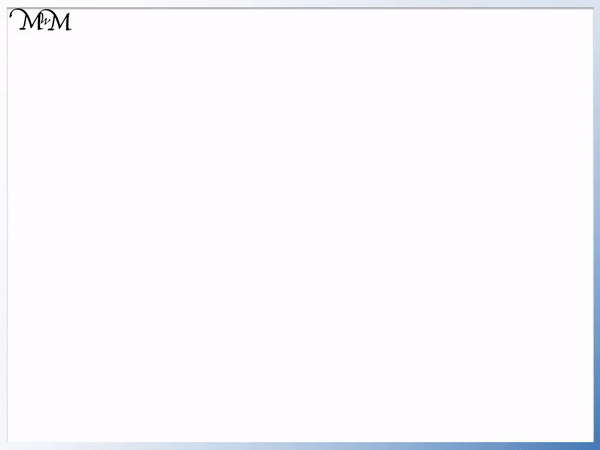We can see that putting the corner of the ruler in the corner of the angle, the sides of the angle line up with the sides of the ruler.

We have a right angle.

To show that we have a right angle, we draw a square in the corner of the angle. This is an easy way to show that we have a right angle without writing 90 degrees next to it.

Here we have a vertical line going up and a horizontal line going left.We can see that the ruler fits exactly in this angle, with the two sides of the angle lining up with the sides of the ruler.

This too is a right angle, so we mark it with a square.

In this example we have a vertical line down and a horizontal line right.

Whenever a vertical line meets a horizontal line, a right angle is formed.

Vertical lines and horizontal lines always meet at right angles.We mark the angle with a square to show that it is a right angle.

Here we have a vertical line down and a horizontal line left.

Again because we have a vertical line meeting a horizontal line, we have another right angle.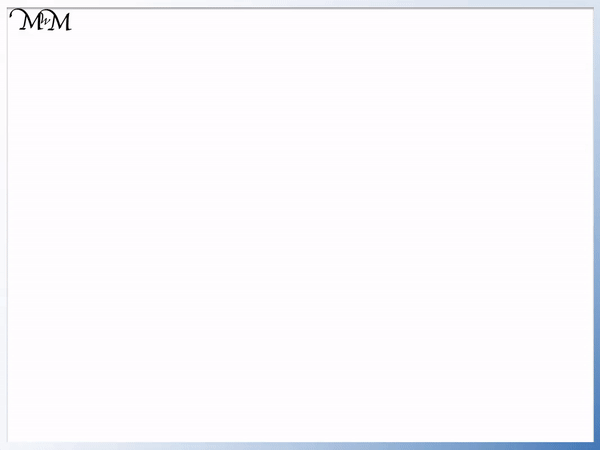The sides of the ruler line up exactly with the two sides of the angle.

In this next example we have a vertical down line but the other line is not horizontal.

We can place on side of the ruler along one of the lines.

The other line does not line up with any of the other sides of the ruler.

These two lines do not form a right angle. We do not mark it with a square box.This angle was too small to be a right angle.

In this next example we have a horizontal line going right and another line.

We can line up one side of the ruler with the horizontal line. We can see that the other line does not line up with the other side of the ruler.Because the sides of the angle do not line up with the sides of the ruler, this is not a right angle. We do not mark it with a square box.

The angle is too large to be a right angle. This means that the angle is too open.

We can see that horizontal and vertical lines are at right angles to each other. If one line is vertical, then the other line must be horizontal to make a right angle. If one line is horizontal, then the other line must be vertical to make a right angle with it.

Right angles do not have to be formed from horizontal and vertical lines. Right angles can face in any direction.

## Examples of Right Angles

Right angles look like capital L shapes. Some common examples of right angles include corners of tables, windows and doors. In the home, some examples of right angles include the corners of dice, books and paper sheets. The corners of a square are all right angles.

Here are some examples of right angles shown going in different directions.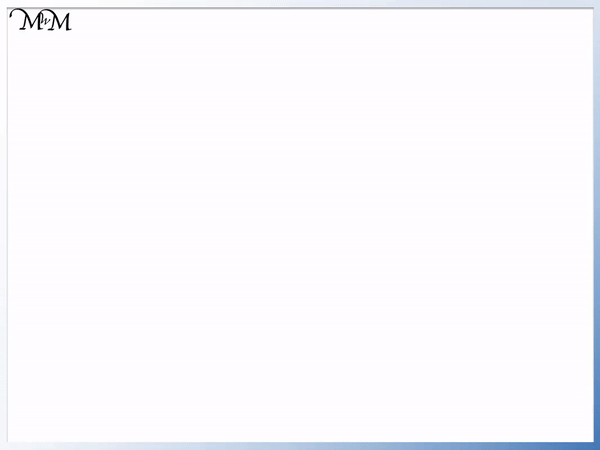Here are some examples of right angles found in real life.

Examples of right angles in real life include the corners of a road sign, the corners of a desk, the corners of a soccer pitch, the corners of a building and crossroads.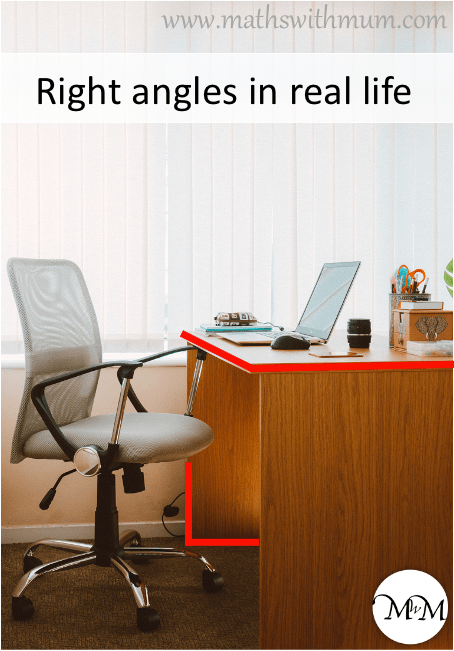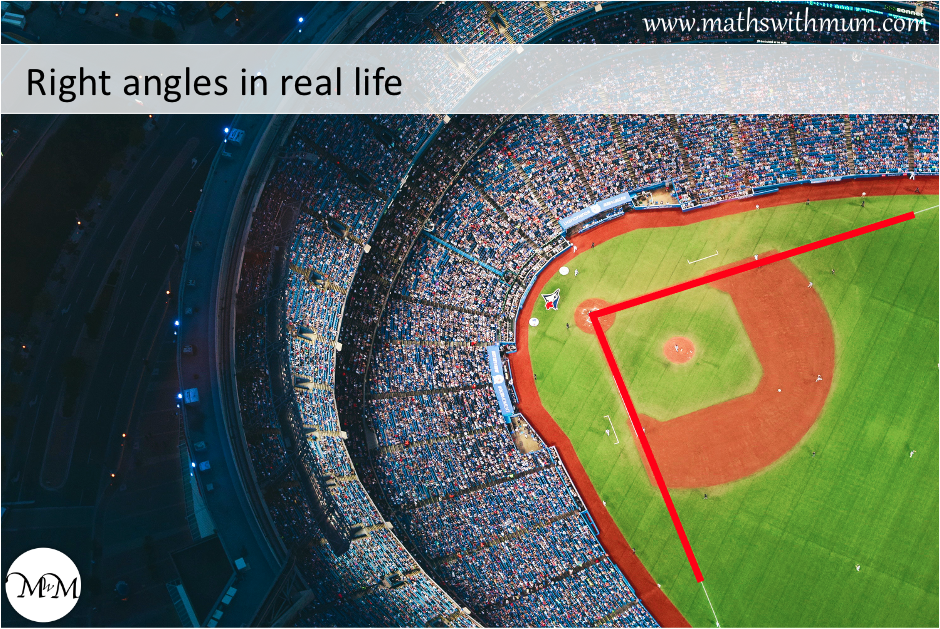Here we can see how these right angles are marked.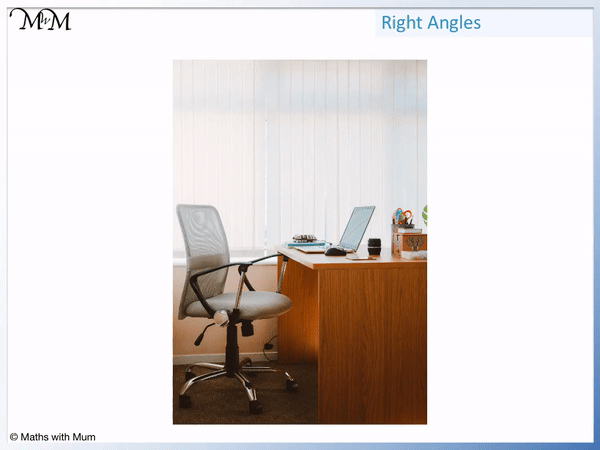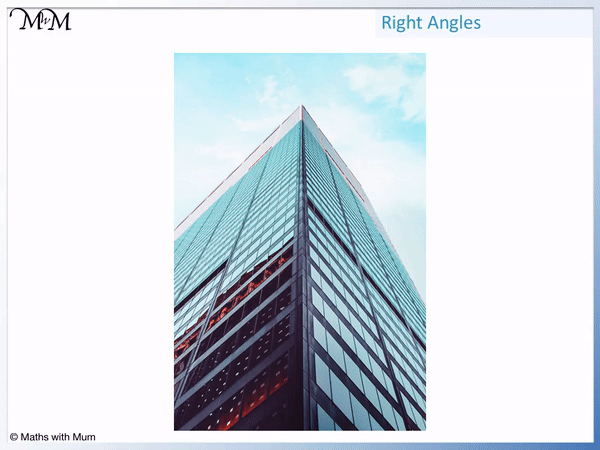## Examples of Right Angles in Nature

Examples of right angles in nature include the angle between trees and the ground, rock formations and the angles between stalactites in caves and the ceiling of the cave.Right angles often form in nature due to the effects of gravity. Gravity acts at right angles to the ground. It pulls things down vertically to make a right angle with the horizontal ground.

We can see the right angles formed in this rock formation.## Examples of Right Angles at Home

Examples of right angles are found all around the home wherever there are horizontal and vertical corners. Examples of right angles around the home include the corners of tables, mats, doors, bricks, windows, television screens, books, folders, boxes and dice.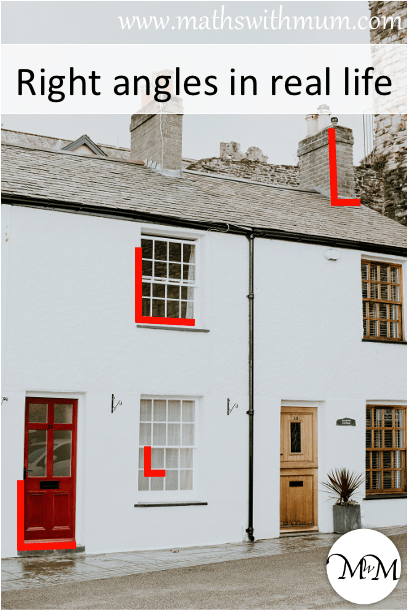Most objects with corners can be tested for right angles. Many objects around the home contain right angles for their corners.

Here are examples of testing corners for right angles.## Right Angles in Shapes

Common shapes that contain right angles are squares, rectangles, right-angled triangles and right trapeziums. The angles of a rhombus and a kite do not typically have right angles but their diagonals will always cross at right angles.

Right angles are often identified as capital L shapes.

Here are some right angles, which can be used to create shapes.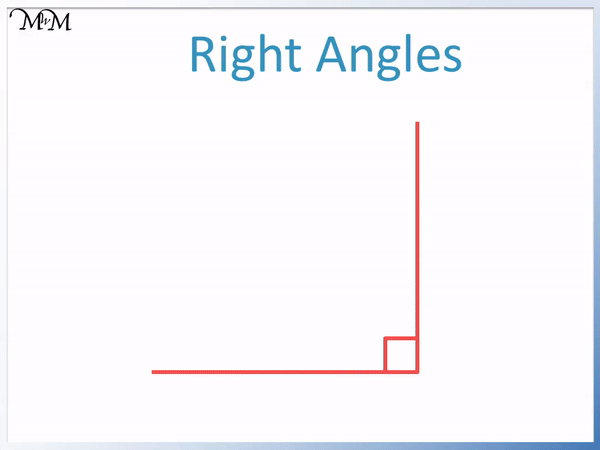Here we can see that four right angles can be used to create a square shape.

To identify right angles in shapes we look for two sides which form a capital L shape. We can line up our ruler to check.

If the sides of the shape line up with the sides of the ruler, the angle is a right angle.

We also look out for horizontal lines meeting vertical lines.

## Right angles on a Square

All four angles on a square are always right angles, meeting at 90 degrees. The four right angles add to make a total of 360 degrees, so the angles in a square add up to 360 degrees.

Below is a square.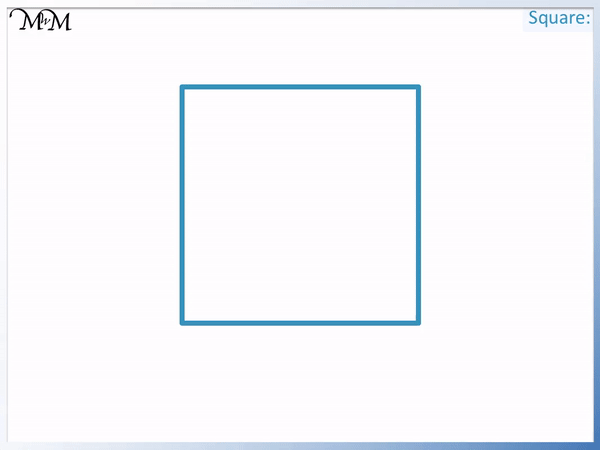We can line the ruler up with the sides of the shape to test for right angles.

We can see that the ruler lines up with the sides at every angle and so, all of the angles in a square are right angles.

A square has 4 right angles and we mark them with a square box.

## Right Angles on a Rectangle

A rectangle always contains 4 right angles. Every angle on a rectangle is 90 degrees. A square is a special type of rectangle that has the same length sides and it also contains 4 right angles.

Below is a rectangle.

We can see that a rectangle also has 4 right angles.## Right Angles on a Triangle

Not all triangles contain right angles. A triangle can contain at most only one right angle and if it does, it is called a right angled triangle. The right angle is shown with a small square in the corner of the angle.

Below is a right-angled triangle.

Only one angle has sides that line up with the sides of the ruler.

This triangle has 1 right angle. The other two angles are too small to be right angles.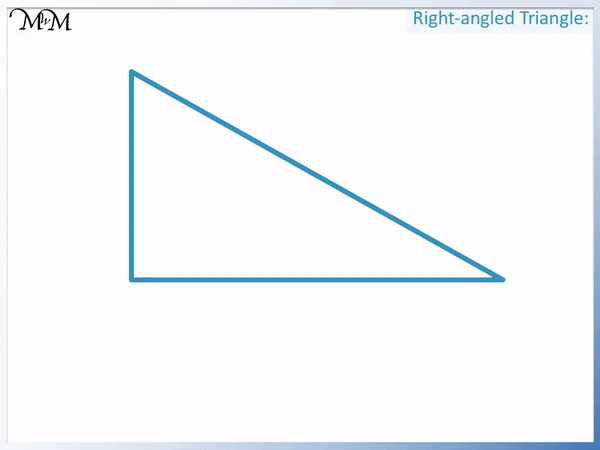We call any triangle that contains a right angle a right-angled triangle.

A triangle can only contain at most one right angle.

Sometimes we don’t have any right angles in a triangle.

The triangle below does not have any right angles.## Right Angles on a Trapezoid

Not all trapezoids contain right angles. Isosceles trapezoids are symmetrical and do not contain any right angles. If a trapezoid contains a right angle, it is called a right trapezoid. In this case, it will have two adjacent right angles connecting the 2 parallel sides.

Below is a trapezoid.

We can see that the two base angles are both right angles. The base is horizontal and the left and right sides are both vertical. Vertical lines that meet horizontal lines create right angles.

The other two angles are not right angles. One angle is too large and the other is too small.## Shapes that do not have a Right Angle

Common shapes that do not contain any right angles include equilateral triangles, regular pentagons, hexagons, octogons. Kites do not always contain right angles, although they can do. Parallelograms do not have to contain right angles, although if they do, they are called rectangles.

Below is a regular pentagon.

All of this pentagon’s angles are the same size.

None of the angles are right angles.Although it is close, the sides of each angle do not line up with the sides of the ruler. The angles are all slightly too large.

Other shapes that don’t have right angles can include kites and parallelograms.Now try our lesson on Parallel Sides where we learn how to identify parallel sides on shapes.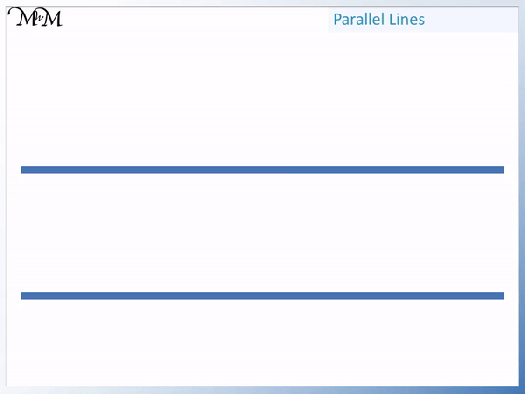error: Content is protected !!